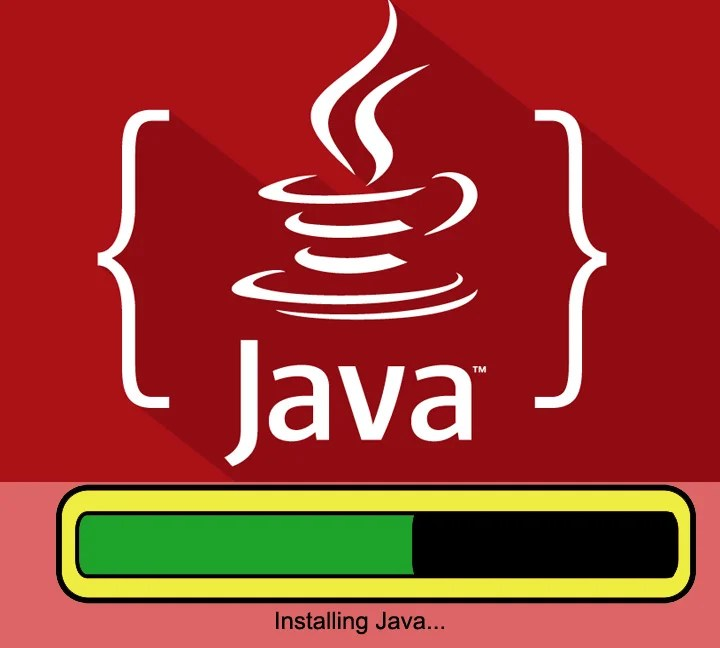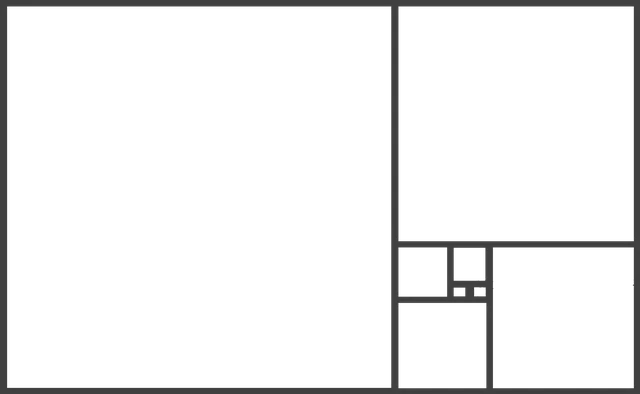Select PageTo code in Java, you need Java Development Kit (JDK). JDK is also known as “Java Platform, Standard Edition” or simply Java SE. The JDK or Java Development Kit can be freely downloaded from official website of Sun Microsystems which is now very much the part of...## Java Programming Source Code – Fibonacci Series

Objective:Write a Program using a JAVA code that accepts a number ‘n’ from the user and generates a Fibonacci series for ‘n’ terms: Fibonacci Series have been around and it is one of the common program that while learning programming every one...## What is fibonacci series?

We often give students a program to write – “To generate a Fibonacci series or Fibonacci Numbers” up to a given no. We need to understand what exactly is Fibonacci series? What is its importance in our lives? Is there any or not? What is Fibonacci...## Java Programming – ETCS-307 Source Code – Practice Programs 1

Objective: Using a JAVA code, find the number of decreasing sequences in the array and also find the length of its longest decreasing sequence: Suppose the array has the following elements: 9 8 7 8 2 8 6 4 2 9 8 5 In this array following decreasing order sequences can...